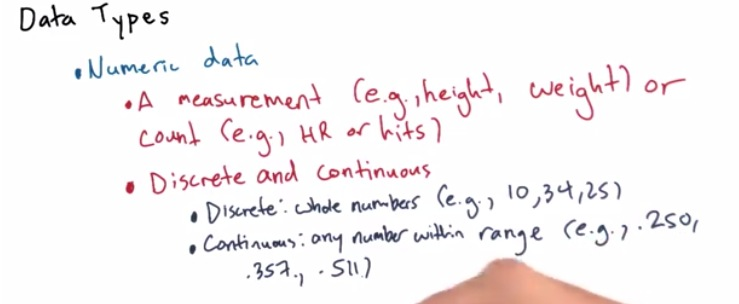• We have seen earlier discussion about numeric being one of data types
• It can represent number of population, or some kind of measurement
• Statistician call it as quantitative data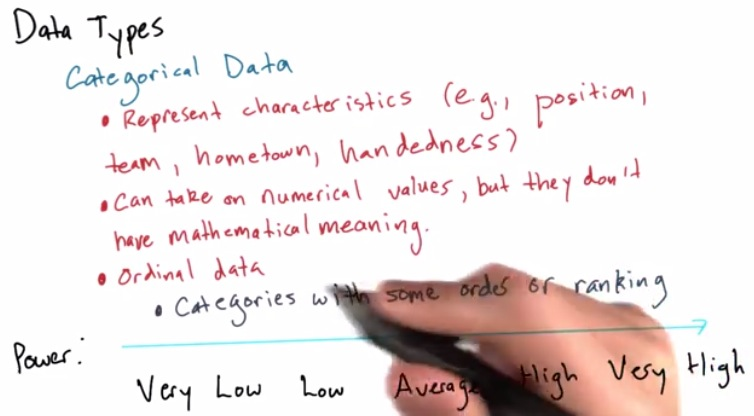• It can differentiate type of groups
• Can't be manipulated, computed, take into calculation, just as character.
• There's also ordinal data, that can be based on ranking or an order.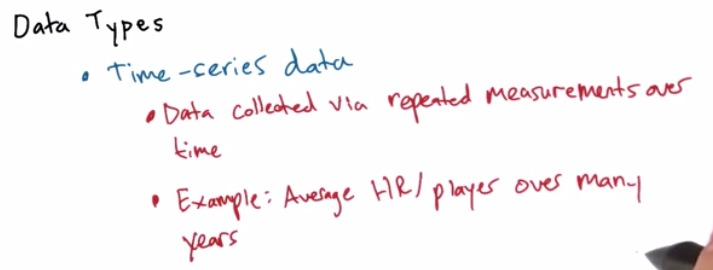• Similar as numerical data. But it was some linear increasing with the data
• There's different about when the data are being collected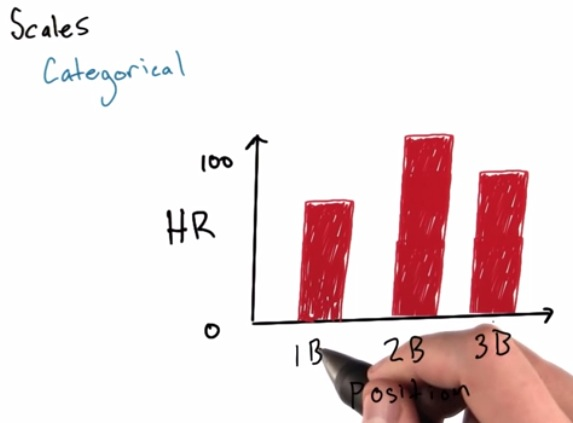• We use numerical data as y-axis and categorical data at x-axis. In this, we sorting (x being ordinal) and rank it so it can be easier to to visualize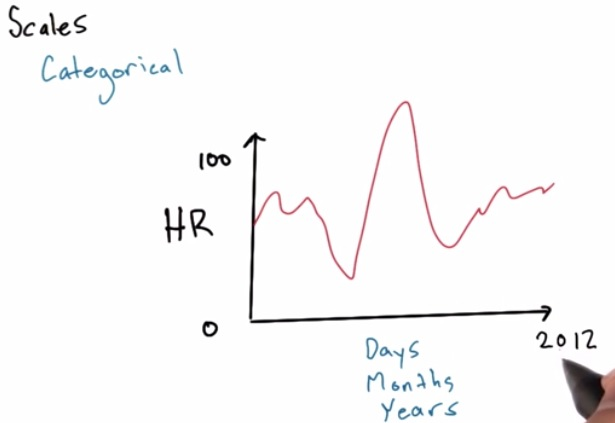• There's also short trend if we want it days-axis, or much longer trends then represent it as month/years axis• This is inapropriate use of scales
• The increasing is random and not linear/logaritmic
• The increasing to pile of people (3d -> 4th) only 3k increasing